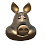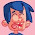### Blender add-on: mesh to heightmap

Sometimes it would be convenient to convert a mesh to a heightmap. If you would have a heightmap available, you could flatten your mesh geometry and add a displacement modifier or material with micro displacement instead.

This has many possibilities, including post processing the heightmap in an external program or using adaptive subdivision to reduce geometric complexity where it isn't needed. Therefore I created a small add-on that does just that: Given a mesh that is uv-mapped it creates a new image which contains the z coordinates as grey-scale values.

(original mesh on the left, flattened mesh with map added via a displacement modifier on the right)

(a uv-mapped plane with a single face plus an adaptive subdivision modifier)

## Limitations

The add-on will map the object z-coord to a grey-scale value, so this will only work properly on rectangular landscapes, not for example spherical ones. The whole range of z-coordinates present will be mapped to the range [0,1].
You can generate any size map you like, even non-square ones, but the map should be smaller than twice the number of vertices in any dimension, and if you select a map size larger than the number of vertices in a dimension you should check the interpolate option to avoid black lines.
For example, if you have generated a 128 x 128 landscape, you can generate a 128 x 128 map, or any smaller one, but for bigger ones up to 256x256 you need to check the interpolate option.
If you need even bigger maps, generate one that fits will and resize in an external program like Gimp.

The add-on is not super robust and will fail if the mesh has no active uv-map and also when the uv-coordinates are outside the range [0,1] (which is perfectly legal but either scale your uv map before generating the heightmap or adapt the add-on)

## Relevant bits of code

For those interested in the inner workings:

• line 05: create a new image
• line 08: initialize the image to an opaque all black
• line 11: get all uv coordinates from the active uv-layer into a numpy array
• line 16: get all vertex coordinates into a numpy array
• line 21: get all vertex indices from the loops. Note that loops and uv-coordinates are aligned, i.e. eery loop has a uv coordinate in a uv-layer
• line 24: scale/map the z component of the coordinates to the range [0,1]
• line 31: map uv-coordinates to image pixel coordinates
• line 35: for every uv value and height, assign the corresponding pixel rgb value. We do not change the alpha. Note that we do this for every loop, not just for every vertex, so this is very inefficient: a typical all-quad mesh contains four loops for every vertex! However, this is fast enough in practice so I didn't bother to optimize
• line 38: optional interpolation. We determine the missing pixel coordinates and then interpolate those values from the immediate neighbors.
• line 50: assign the calculated grey-scale values to the pixels. Note that copying a complete array like we do here is rather fast but would we have done it by indexing the pixels attribute pixel by pixel this would have been prohibitively slow.

``` def execute(self, context):
mesh = context.active_object.data
width, height = self.width, self.height

im = bpy.data.images.new("Heightmap",
height, width, float_buffer=True)

hm = np.zeros((width, height, 4), dtype=np.float32)
hm[:,:,3] = 1.0

uvlayer = mesh.uv_layers.active.data
uv = np.empty(len(uvlayer)*2, dtype=np.float32)
uvlayer.foreach_get('uv',uv)
uv.shape = -1,2

co = np.empty(len(mesh.vertices)*3, dtype=
np.float32)
mesh.vertices.foreach_get('co', co)
co.shape = -1,3

vi = np.empty(len(mesh.loops), dtype=np.int32)
mesh.loops.foreach_get('vertex_index',vi)

z = co[:,2][vi]
zmax = np.max(z)
zmin = np.min(z)
zd = zmax - zmin
z -= zmin
z /= zd

uv[:,0] *= height-1
uv[:,1] *= width-1
uv = np.round(uv).astype(np.int32)

for h,xy in zip(z,uv):
hm[xy,xy,:3] = h

if self.interpolate:
uniq0 = np.unique(uv[:,0])
uniq1 = np.unique(uv[:,1])
missing0 = np.setdiff1d(np.arange(height,
dtype=np.int32), uniq0)
missing1 = np.setdiff1d(np.arange(height,
dtype=np.int32), uniq1)
for y in missing1:
hm[y,:] = (hm[y-1,:] + hm[y-1,:])/2
for x in missing0:
hm[:,x] = (hm[:,x-1] + hm[:,x+1])/2

im.pixels[:] = hm.flat[:]
```

## Availability

The add-on is available from my GitHub repository (right click on link and select Save As ... or equivalent for your browser). After installing and enabling the add-on, a new menu item `Object -> Mesh2Heightmap` will be available in the 3d-view in object mode.

1.Hello! I tried this out and I get an index out of range error at line 103 (hm[xy,xy,:3] = h). Do you know what it could be?

2.Not sure, most likely your uvmap contains values outside 0,1 range

3.Hi. I have tried, but I don't understand how it work. PLease could you provide a simple exemple ? What I have tried (in blender 2.79) : - create a plane, subdivide it in 10x10 (or 16x16), create a uvmapping with unwrap, add a new image to the mesh (in th uveditor), then clic on object and select mesh2heighmap, and nothing appears. where is the image of heighmap ? Thank you for you answer.

1.it creates a new Image object and this should be visible in an image/texture viewer window (you may have to open one if your workspace does have one open already). You may have to select it from the dropdown if the viewer shows a different image/texture. The default name will be 'Heightmap'